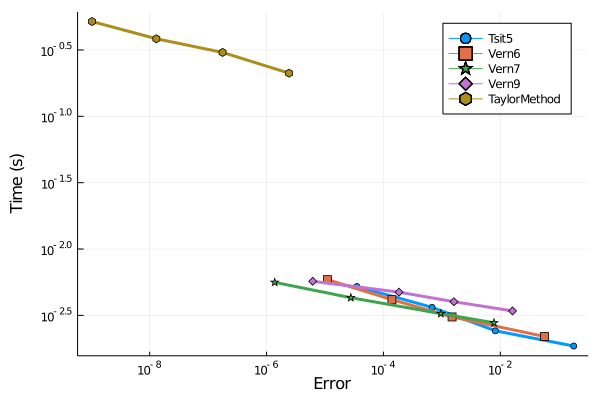{{ message }}

Instantly share code, notes, and snippets.

# Christopher Rackauckas ChrisRackauckas

🎯
Focusing
Created Jun 22, 2021
hasbranching for automatically specializing ReverseDiff tape compilation
View hasbranching.jl
This file contains bidirectional Unicode text that may be interpreted or compiled differently than what appears below. To review, open the file in an editor that reveals hidden Unicode characters. Learn more about bidirectional Unicode characters
 using Cassette, DiffRules using Core: CodeInfo, SlotNumber, SSAValue, ReturnNode, GotoIfNot const printbranch = true Cassette.@context HasBranchingCtx function Cassette.overdub(ctx::HasBranchingCtx, f, args...) if Cassette.canrecurse(ctx, f, args...) return Cassette.recurse(ctx, f, args...)
Last active Dec 6, 2021
DiffEqFlux.jl (Julia) vs Jax on an Epidemic Model
View diffeqflux_vs_jax_results.md

# DiffEqFlux.jl (Julia) vs Jax on an Epidemic Model

The Jax developers optimized a differential equation benchmark in this issue which used DiffEqFlux.jl as a performance baseline. The Julia code from there was updated to include some standard performance tricks and is the benchmark code here. Thus both codes have been optimized by the library developers.

## Results

### Forward Pass

Created Mar 11, 2021
Symbolics.jl Lotka-Volterra Tracing result
View gist:c05f580c40378dd6da2274e61fca599a
This file contains bidirectional Unicode text that may be interpreted or compiled differently than what appears below. To review, open the file in an editor that reveals hidden Unicode characters. Learn more about bidirectional Unicode characters
 retcode: Success Interpolation: 3rd order Hermite t: [0.0, 0.5, 1.0] u: Vector{Num}[[x0, y0], [x0 + 0.08333333333333333((1.5x0) + (1.5(x0 + (0.5((1.5(x0 + (0.25((1.5(x0 + (0.25((1.5x0) - (x0*y0))))) - ((x0 + (0.25((1.5x0) - (x0*y0))))*(y0 + (0.25((x0*y0) - (3y0))))))))) - ((x0 + (0.25((1.5(x0 + (0.25((1.5x0) - (x0*y0))))) - ((x0 + (0.25((1.5x0) - (x0*y0))))*(y0 + (0.25((x0*y0) - (3y0))))))))*(y0 + (0.25(((x0 + (0.25((1.5x0) - (x0*y0))))*(y0 + (0.25((x0*y0) - (3y0))))) - (3(y0 + (0.25((x0*y0) - (3y0))))))))))))) + (2((1.5(x0 + (0.25((1.5x0) - (x0*y0))))) + (1.5(x0 + (0.25((1.5(x0 + (0.25((1.5x0) - (x0*y0))))) - ((x0 + (0.25((1.5x0) - (x0*y0))))*(y0 + (0.25((x0*y0) - (3y0))))))))) - ((x0 + (0.25((1.5x0) - (x0*y0))))*(y0 + (0.25((x0*y0) - (3y0))))) - ((x0 + (0.25((1.5(x0 + (0.25((1.5x0) - (x0*y0))))) - ((x0 + (0.25((1.5x0) - (x0*y0))))*(y0 + (0.25((x0*y0) - (3y0))))))))*(y0 + (0.25(((x0 + (0.25((1.5x0) - (x0*y0))))*(y0 + (0.25((x0*y0) - (3y0))))) - (3(y0 + (0.25((x0*y0) - (3y0))))))))))) - (x0*y0) - ((x0 + (0.5(
Last active Nov 4, 2020
Lotka-Volterra Learned via a Neural ODE (takes about 1 minute!)
View lotka_volterra_neural_ode.jl
This file contains bidirectional Unicode text that may be interpreted or compiled differently than what appears below. To review, open the file in an editor that reveals hidden Unicode characters. Learn more about bidirectional Unicode characters
 using OrdinaryDiffEq using Plots using Flux, DiffEqFlux, Optim function lotka_volterra(du,u,p,t) x, y = u α, β, δ, γ = p du = dx = α*x - β*x*y du = dy = -δ*y + γ*x*y end
Created Sep 27, 2020
State-dependent delay diffeq model from "Modelling the Effects of Temperature on Temperature Mosquito Seasonal Abundance"
View ewing-optim-cr.jl
This file contains bidirectional Unicode text that may be interpreted or compiled differently than what appears below. To review, open the file in an editor that reveals hidden Unicode characters. Learn more about bidirectional Unicode characters
 # -------------------------------------------------------------------------------- # Ewing model # translation by: slwu89@berkeleu.edu (July 2020) # -------------------------------------------------------------------------------- using DifferentialEquations using Plots using LabelledArrays using StaticArrays
Created Sep 6, 2020
Conservative package compiler testing
View conservative_package_compiler_results.md

## Conservative Package Compiler Results

1. Using a ton of standard pervasive packages only helps 10%
2. Getting DiffEqBase helps 50%
3. Getting OrdinaryDiffEq without extra precompiles gets to 1.3 seconds, vs 0.6 seconds on the second run. That's pretty good!
Last active Aug 31, 2020
DifferentialEquations.jl Runge-Kutta integrators vs Taylor Integration Benchmarks
View differential_equations_taylor_integration_benchmark.md

# DifferentialEquations.jl Runge-Kutta Integrators vs Taylor IntegrationThis is against a Taylor integration method that utilizes taylor-mode automatic differentiation in a specifically optimized way for ODE integration via its `@taylorize` macro (for more details, see the documentation). This demonstration is done on the classic Pleiades n-body problem, a non-stiff ODE since the Taylor integration methods are only for non-stiff equations.

Last active Dec 4, 2021
torchdiffeq vs Julia DiffEqflux Neural ODE Training Benchmark
View neural_ode_benchmarks.md

# torchdiffeq vs Julia DiffEqFlux Neural ODE Training Benchmark

The spiral neural ODE was used as the training benchmark for both torchdiffeq (Python) and DiffEqFlux (Julia) which utilized the same architecture and 500 steps of ADAM. Both achived similar objective values at the end. Results:

• DiffEqFlux defaults: 7.4 seconds
• DiffEqFlux optimized: 2.7 seconds
• torchdiffeq: 288.965871299999 seconds
Last active Nov 2, 2021
torchsde vs DifferentialEquations.jl / DiffEqFlux.jl (Julia) benchmarks
View diffeq_vs_torchsde.md

# torchsde vs DifferentialEquations.jl / DiffEqFlux.jl (Julia)

This example is a 4-dimensional geometric brownian motion. The code for the torchsde version is pulled directly from the torchsde README so that it would be a fair comparison against the author's own code. The only change to that example is the addition of a `dt` choice so that the simulation method and time step matches between the two different programs.

The SDE is solved 100 times. The summary of the results is as follows:

Created Jul 25, 2020
Automated multithreaded sparse Jacobians in Julia via ModeingToolkit.jl